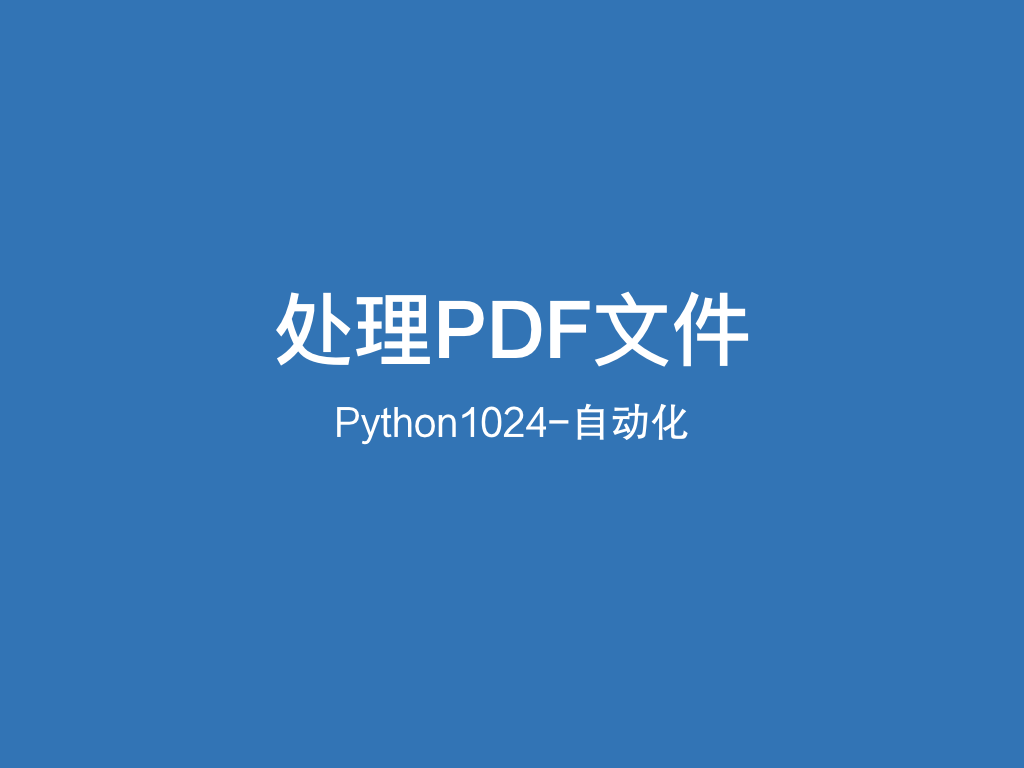# Python 处理 PDF 的实用姿势# ﻿

﻿

PDF文件我们经常用，尤其是这两个场景：

﻿

• 下载参考资料，如各类报告、文档

• 分享只读资料，方便传播同时保留源文件

﻿

## 场景和模块

﻿

﻿

• 处理文件本身，属于文件页面级操作，如合并/分拆PDF页面、加/解密、加/去水印；

• 处理文件内容，属于内容级操作，如提取文字、表格数据、图表等。

﻿

﻿

• PyPDF2：模块成熟，最后一次更新在2年前，适合页面级操作，文字提取效果较差。

• PDFMiner：擅长文字抽取，目前主分支已停止维护，取而代之的是pdfminer.six

• pdfplumber：基于pdfminer.six的文本内容抽取工具，使用门槛更低，如支持表格提取。

﻿

﻿

﻿

• pip install pypdf2

• pip install pdfminer.six

• pip install pdfplumber

﻿

﻿

## PyPDF2

﻿

PyPDF2的主要能力在页面级操作，比如：

﻿

• 获取PDF文档基本信息

• PDF分割及合并

• PDF的旋转及排序

• PDF加水印及去水印

• PDF加密及解密

﻿

PyPDF2的核心两个类是PdfFileReaderPdfFileWriter，完成PDF文件的读写操作。

﻿

### 获取PDF文档基本信息

﻿

import pathlibfrom PyPDF2 import PdfFileReaderpath = list(pathlib.Path.cwd().parents).joinpath('data/automate/002pdf')f_path = path.joinpath('2020-新冠肺炎疫情对中国连锁餐饮行业的影响调研报告-中国连锁经营协会.pdf')with open(f_path, 'rb') as f:    pdf = PdfFileReader(f)    info = pdf.getDocumentInfo()    cnt_page = pdf.getNumPages()    is_encrypt = pdf.getIsEncrypted()print(f'''作者: {info.author}创建者: {info.creator}制作者: {info.producer}主题: {info.subject}标题: {info.title}总页数: {cnt_page}是否加密: {is_encrypt}''')

﻿

### PDF分割及合并

﻿

import pathlibfrom PyPDF2 import PdfFileReader, PdfFileWriterpath = list(pathlib.Path.cwd().parents).joinpath('data/automate/002pdf')f_path = path.joinpath('2020-新冠肺炎疫情对中国连锁餐饮行业的影响调研报告-中国连锁经营协会.pdf')out_path = path.joinpath('002pdf_split_merge.pdf')out_path_1 = path.joinpath('002pdf_split_half_front.pdf')out_path_2 = path.joinpath('002pdf_split_half_back.pdf')# 把文件分为两半with open(f_path, 'rb') as f, open(out_path_1, 'wb') as f_out1, open(out_path_2, 'wb') as f_out2:    pdf = PdfFileReader(f)    pdf_out1 = PdfFileWriter()    pdf_out2 = PdfFileWriter()    cnt_pages = pdf.getNumPages()    print(f'共 {cnt_pages} 页')    for i in range(cnt_pages):        if i <= cnt_pages //2:            pdf_out1.addPage(pdf.getPage(i))        else:            pdf_out2.addPage(pdf.getPage(i))    pdf_out1.write(f_out1)    pdf_out2.write(f_out2)# 再把后半个文件与前半个文件合并，后半个文件在前with open(out_path, 'wb') as f_out:    cnt_f, cnt_b = pdf_out1.getNumPages(), pdf_out2.getNumPages()    pdf_out = PdfFileWriter()    for i in range(cnt_b):        pdf_out.addPage(pdf_out2.getPage(i))    for i in range(cnt_f):        pdf_out.addPage(pdf_out1.getPage(i))    pdf_out.write(f_out)

﻿

### PDF的旋转及排序

﻿

import pathlibfrom PyPDF2 import PdfFileReader, PdfFileWriterpath = list(pathlib.Path.cwd().parents).joinpath('data/automate/002pdf')f_path = path.joinpath('2020-新冠肺炎疫情对中国连锁餐饮行业的影响调研报告-中国连锁经营协会.pdf')out_path = path.joinpath('002pdf_rotate.pdf')with open(f_path, 'rb') as f, open(out_path, 'wb') as f_out:    pdf = PdfFileReader(f)    pdf_out = PdfFileWriter()    page = pdf.getPage(0).rotateClockwise(90)    pdf_out.addPage(page)    # 把第二页放到前面    pdf_out.addPage(pdf.getPage(2))    page = pdf.getPage(1).rotateCounterClockwise(90)    pdf_out.addPage(page)    pdf_out.write(f_out)

﻿

### PDF加水印及去水印

﻿

﻿

import pathlibfrom PyPDF2 import PdfFileReader, PdfFileWriterpath = list(pathlib.Path.cwd().parents).joinpath('data/automate/002pdf')f_path = path.joinpath('2020-新冠肺炎疫情对中国连锁餐饮行业的影响调研报告-中国连锁经营协会.pdf')wm_path = path.joinpath('watermark.pdf')en_path = path.joinpath('002pdf_with_watermark_en.pdf')out_path = path.joinpath('002pdf_with_watermark.pdf')with open(f_path, 'rb') as f, open(wm_path, 'rb') as f_wm, open(out_path, 'wb') as f_out:    pdf = PdfFileReader(f)    pdf_wm = PdfFileReader(f_wm)    pdf_out = PdfFileWriter()    wm_cn_page = pdf_wm.getPage(0)    wm_en_page = pdf_wm.getPage(1)    cnt_pages = pdf.getNumPages()    for i in range(cnt_pages):        page = pdf.getPage(i)        page.mergePage(wm_cn_page)        pdf_out.addPage(page)    pdf_out.write(f_out)

﻿

﻿

1. 找到特征词后替换，适合英文文档，但不适用于中文等CJK字符。

2. 把PDF页转成图片后，用图像算法去水印，但这样会破坏文件原信息结构。

3. 根据水印大小位置特征，找到所有元素后删除。这是更推荐的方式。

﻿

﻿

﻿

### PDF加密解密

﻿

PDF里的密码，分为用户密码和所有者密码。

PyPDF2里提供了基本的加密功能，“防君子不防小人”。

﻿

import pathlibfrom PyPDF2 import PdfFileReader, PdfFileWriterpath = list(pathlib.Path.cwd().parents).joinpath('data/automate/002pdf')f_path = path.joinpath('2020-新冠肺炎疫情对中国连锁餐饮行业的影响调研报告-中国连锁经营协会.pdf')out_path_encrypt = path.joinpath('002pdf_encrypt.pdf')out_path_decrypt = path.joinpath('002pdf_decrypt.pdf')with open(f_path, 'rb') as f, open(out_path_encrypt, 'wb') as f_out:    pdf = PdfFileReader(f)    pdf_out = PdfFileWriter()    cnt_pages = pdf.getNumPages()    for i in range(cnt_pages):        page = pdf.getPage(i)        pdf_out.addPage(page)    pdf_out.encrypt('123456', owner_pwd='654321')    pdf_out.write(f_out)# 重新读取加密文件并生成解密文件with open(out_path_encrypt, 'rb') as f, open(out_path_decrypt, 'wb') as f_out:    pdf = PdfFileReader(f)    if not pdf.isEncrypted:        print('文件未被加密')    else:        success = pdf.decrypt('123456')        # if not success:        pdf_out = PdfFileWriter()        pdf_out.appendPagesFromReader(pdf)        pdf_out.write(f_out)

﻿

## pdfminer.six

﻿

PDFMiner的操作门槛比较高，需要部分了解PDF的文档结构模型，适合定制开发复杂的内容处理工具。

﻿

﻿

import pathlibfrom pdfminer.pdfparser import PDFParserfrom pdfminer.pdfdocument import PDFDocumentfrom pdfminer.pdfpage import PDFPagefrom pdfminer.pdfinterp import PDFResourceManagerfrom pdfminer.pdfinterp import PDFPageInterpreterfrom pdfminer.pdfdevice import PDFDevicefrom pdfminer.layout import LAParams, LTTextBox, LTFigure, LTImagefrom pdfminer.converter import PDFPageAggregatorpath = list(pathlib.Path.cwd().parents).joinpath('data/automate/002pdf')f_path = path.joinpath('2020-新冠肺炎疫情对中国连锁餐饮行业的影响调研报告-中国连锁经营协会.pdf')with open(f_path, 'rb') as f:    parser = PDFParser(f)    doc = PDFDocument(parser)    rsrcmgr = PDFResourceManager()    laparams = LAParams()    device = PDFPageAggregator(rsrcmgr, laparams=laparams)    interpreter = PDFPageInterpreter(rsrcmgr, device)    for page in PDFPage.create_pages(doc):        interpreter.process_page(page)        layout = device.get_result()        for x in layout:            # 获取文本对象            if isinstance(x, LTTextBox):                print(x.get_text().strip())            # 获取图片对象            if isinstance(x,LTImage):                print('这里获取到一张图片')            # 获取 figure 对象            if isinstance(x,LTFigure):                print('这里获取到一个 figure 对象')

﻿

﻿

## pdfplumber

﻿

﻿

﻿

• 提取PDF内容，保存到txt文件

• 提取PDF中的表格到Excel

• 提取PDF中的图片

• 提取PDF中的图表

### 提取PDF内容，保存到txt文件

﻿

import pathlibimport pdfplumberpath = list(pathlib.Path.cwd().parents).joinpath('data/automate/002pdf')f_path = path.joinpath('2020-新冠肺炎疫情对中国连锁餐饮行业的影响调研报告-中国连锁经营协会.pdf')out_path = path.joinpath('002pdf_out.txt')with pdfplumber.open(f_path) as pdf, open(out_path ,'a') as txt:    for page in pdf.pages:        textdata = page.extract_text()        txt.write(textdata)

﻿

### 提取PDF中的表格到Excel

﻿

import pathlibimport pdfplumberfrom openpyxl import Workbookpath = list(pathlib.Path.cwd().parents).joinpath('data/automate/002pdf')f_path = path.joinpath('2020-新冠肺炎疫情对中国连锁餐饮行业的影响调研报告-中国连锁经营协会.pdf')out_path = path.joinpath('002pdf_excel.xlsx')wb = Workbook()sheet = wb.activewith pdfplumber.open(f_path) as pdf:    for i in range(19, 22):        page = pdf.pages[i]        table = page.extract_table()        for row in table:            sheet.append(row)wb.save(out_path)

﻿

﻿

### 提取PDF中的图片

﻿

import pathlibimport pdfplumberfrom PIL import Imagepath = list(pathlib.Path.cwd().parents).joinpath('data/automate/002pdf')f_path = path.joinpath('2020-疫情影响下的中国社区趋势研究-艾瑞.pdf')out_path = path.joinpath('002pdf_images.png')with pdfplumber.open(f_path) as pdf, open(out_path, 'wb') as fout:    page = pdf.pages    # for img in page.images:    im = page.to_image()    im.save(out_path, format='PNG')    imgs = page.images    for i, img in enumerate(imgs):        size = img['width'], img['height']        data = img['stream'].get_data()        out_path = path.joinpath(f'002pdf_images_{i}.png')        with open(out_path, 'wb') as fimg_out:            fimg_out.write(data)

﻿

### 提取PDF中的图表

﻿

﻿

import pathlibimport pdfplumberfrom PIL import Imagepath = list(pathlib.Path.cwd().parents).joinpath('data/automate/002pdf')f_path = path.joinpath('2020-新冠肺炎疫情对中国连锁餐饮行业的影响调研报告-中国连锁经营协会.pdf')out_path = path.joinpath('002pdf_figures.png')with pdfplumber.open(f_path) as pdf, open(out_path, 'wb') as fout:    page = pdf.pages    im = page.to_image()    im.save(out_path, format='PNG')    figures = page.figures    for i, fig in enumerate(figures):        size = fig['width'], fig['height']        crop = page.crop((fig['x0'], fig['top'], fig['x1'], fig['bottom']))        img_crop = crop.to_image()        out_path = path.joinpath(f'002pdf_figures_{i}.png')        img_crop.save(out_path, format='png')    im.draw_rects(page.extract_words(), stroke='yellow')    im.draw_rects(page.images, stroke='blue')    im.draw_rects(page.figures)im # show in notebook

﻿

## 总结

﻿

﻿

﻿

﻿

﻿

﻿﻿## 评论